Sponsored Link •Weblogs Forum
The Adventures of a Pythonista in Schemeland/14

2 replies on 1 page. Most recent reply: Feb 6, 2009 8:49 PM by Michele Simionato

 Welcome Guest   Sign In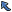Back to Topic List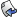Reply to this Topic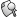Search Forum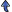Threaded View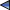Previous Topic Next Topic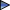Flat View: This topic has 2 replies on 1 page
 Michele Simionato Posts: 222 Nickname: micheles Registered: Jun, 2008
The Adventures of a Pythonista in Schemeland/14 (View in Weblogs)
Posted: Feb 4, 2009 10:51 PMReply
Summary
Everything you ever wanted to know about currying, partial application, higher order functions and related topics.
 Advertisement# Higher order functions and curried functions

A language has support for first class functions if it is possible to use a function as a regular value, i.e. if it is possible to pass a function to another function, or return it from a function. In a language with first class functions, it is therefore possible to define the concept of higher order function is: a function which accepts in input or returns in output (or both) another function.

Various imperative languages have support for higher order functions: all the scripting languages, the latest version of C#, Scala, and a few others. Still, functional languages have a better support and higher order functions are used in those language much more that in imperative languages. This is especially true for languages such as ML and Haskell, which support curried functions out of the box: in such languages all functions are really unary functions (i.e. they accept a single argument) and functions of n arguments are actually unary functions returning closures. In Scheme this behavior can be emulated with macros. Here is an example of how one could define curried functions in Scheme:

```(def-syntax curried-lambda
(syntax-match ()
(sub (curried-lambda () b b* ...)
#'(begin b b* ...))
(sub (curried-lambda (x x* ...) b b* ...)
#'(lambda (x) (curried-lambda (x* ...) b b* ...)))
))

(def-syntax (define-curried (f x ...) b b* ...)
#'(define f (curried-lambda (x ...) b b* ...)))
```

define-curried defines a function with (apparently) n arguments as an unary function returning a closure, i.e. a function with (apparently) n-1 arguments which in turns is an unary function returning a closure with n-2 arguments and so on, until it returns an unary function. For instance, the following add function

```(define-curried (add x y) (+ x y))
```

apparently has two arguments, but actually it is an unary function returning an unary closure:

```> (add 1)
#<procedure>
> ((add 1) 2)
3
```

You can see how the macro works by using syntax-expand:

```> (syntax-expand (curried-lambda (x y) (+ x y)))
(lambda (x) (curried-lambda (y) (+ x y)))
```

The internal curried-lambda has a single argument in this case and thus expands to a regular lambda function, but you can see that in general you will have a tower of nested lambdas, which dept is equal to the number of arguments.Whereas it is possible define curried functions in Scheme, usually this is not very convenient, unless you are trying to emulate ML or Haskell idioms. Out of the box, Scheme supports functions with multiple arguments in a traditional fashion, i.e. the same as in Python: thus, the most convenient construct is not currying, but partial application. The Pythonistas here will certainly think of functools.partial, an utility which was added to the standard library starting from Python 2.5. Schemers have something similar (but of course better) in the form of SRFI-26, i.e. the cut and cute macros by Al Petrofsky.

# Partial application: cut and cute

Instead of spending too many words, let me show an example of how partial function application works both in Python and in Scheme.

Here is the Python version:

```>>> from functools import partial
>>> from operator import add
>>> add1 = partial(add, 1)
>>> add1(2)
3
```

and here is the Scheme version:

```> (import (srfi-26)); assuming it is available in your implementation
> (define add1 (cut + 1 <>))
> (add1 2)
3
```

In Python, partial(add, 1) returns an unary callable object that adds 1 to its argument; in Scheme, (cut + 1 <>) returns an unary function that does the same. The Scheme version is better, since the arguments of the resulting functions are immediately vas visible as slots (i.e. the <> symbol). For instance

```> (define greetings (cut string-append "hello " <> " and " <>))
```

has two slots and therefore is a function of two arguments:

```> (greetings "Michele" "Mario")
"hello Michele and Mario"
```

It is also possible to define a variable number of arguments by using the rest-slot symbol <...>:

```> (define greetings (cut string-append "hello " <> " and " <...>))
> (display (greetings "Michele" "Mario" "\n"))
hello Michele and Mario
```

We can even use a slot for the function: for instance, the higher order function apply could be implemented as (cut <> <...>).

Moreover, there is a cute macro which acts exactly as cut, with a single difference: the arguments in cute are evalued only once (the e stands for evalued), whereas cut is not safe against multiple evaluation. In particular, if you define

```> (define add-result (cute + (long-computation) <>))
```

then add-result performs the long computation only once, at definition time, and not every time it is called. For more details I refer you the SRFI-26 specification.

# fold-left and fold-right

A couple of commonly used higher order functions in Scheme and other functional languages are fold-left and fold-right. They entered in the R6RS standard, but they are also available from SRFI-1, therefore you can leverage on them even if you are using an R5RS Scheme.

fold-left and fold-right will remind Pythonistas of reduce, which is also a folding function. However, it is well known that Guido dislikes it and nowadays reduce is no more a builtin (in Python 3.0); it is still available in the functools module, though. For some reason (probabily the order of the arguments which I cannot remember) I cannot use reduce in Python, whereas I have less problems with fold-left e fold-right in Scheme and other functional languages.

fold-left and fold-right have a nearly identical API: both allow to traverse a list by accumulating values and by returning at the end the final accumulator. For instance, if you want to sum the values of list, here is an idiomatic solution in Scheme (another one is (apply + numbers-list):

```> (fold-left + 0 '(1 2 3)); sum all elements starting from 0; fold-right works too
6
```

In general, the function in fold-left takes N + 1 arguments, where N is the number of lists you are looping over (usually N = 1) and the leftmost argument is the accumulator. The same is true for fold-right, but then the rightmost argument is the accumulator.

Notice that fold-left is quite different from fold-right, since they work in opposite order:

```> (fold-left (lambda (acc el) (cons el acc)) '() '(1 2 3))
(3 2 1)

> (fold-right (lambda (el acc) (cons el acc)) '() '(1 2 3))
(1 2 3)
```

In the first case fold-left loops from left to right (the element 1 is the first to be consed, the element 2 is the second to be consed, and the element 3 is the last to be consed, so that the final result is (cons 3 (cons 2 (cons 1 '()))) i.e. (3 2 1)) whereas in the second case fold-right loops from right to left.

In order to give an example of use, here is how you could define a flattening procedure by using fold-right:

```(define (flatten lst)
(fold-right
(lambda (x a)
(if (list? x) (append (flatten x) a) (cons x a))) '() lst))
```

You can check that it works with a few tests:

```(test "flatten null"
(flatten '())
'())
(test "flatten plain"
(flatten '(a b c))
'(a b c))
(test "flatten nested"
(flatten '((a b) (c (d e) f)))
'(a b c d e f))
```

Here is another example, a function to remove duplicates from a list:

```;; ex: (remove-dupl = '(1 2 3 1 5 2 4)) => (1 2 3 5 4)
(define (remove-dupl eq? lst)
(reverse
(fold-left
(lambda (acc el)
(if (exists (cut eq? <> el) acc); duplicate
acc
(cons el acc)))
'() lst)))
```

Notice the use of cut to define an unary function (cut eq? <> el) which checks if its argument is equal - according to the provided equality function - to a given element el. exists is one of the list processing utilities standardized by the R6RS document. Here is a test:

```(test "remove-dupl"
(remove-dupl equal? '(1 #f 2 #f 3))
'(1 #f 2 3))
```

Having first class functions in a language means much more than having map, filter or fold. Perhaps in the future I will add another episode about advanced usage of functions, such a parsing combinators or formatting combinators; for the moment what I said here should be enough, and the next episode will be devoted to another typical feature of functional languages: pattern matching.

 Elizabeth Wiethoff Posts: 89 Nickname: ewiethoff Registered: Mar, 2005
Re: The Adventures of a Pythonista in Schemeland/14 Posted: Feb 6, 2009 9:04 AMReply
Cute! It's fun to see examples of similar functions/techniques in different languages. And thanks for the little lesson on Python's `functools.partial`. My Python skills are way behind the times.

> For some reason (probabily the order of the arguments which I cannot remember) I cannot use reduce in Python, whereas I have less problems with fold-left e fold-right in Scheme and other functional languages.

Now I'm curious. Can you say more about your `reduce` problems?

 Michele Simionato Posts: 222 Nickname: micheles Registered: Jun, 2008
Re: The Adventures of a Pythonista in Schemeland/14 Posted: Feb 6, 2009 8:49 PMReply
> For some reason (probabily the order of the arguments
> which I cannot remember) I cannot use reduce in Python,
> whereas I have less problems with fold-left e fold-right
> in Scheme and other functional languages.
>
> Now I'm curious. Can you say more about your
> `reduce` problems?

map and filter in Python have signature map(function, sequence, ...), the same as in Scheme. reduce instead
has a different signature: reduce(binary_op, sequence, seed)
I would expect (as done consistently in Scheme) a
signature reduce(function, seed, sequence, ...) so I
get confused all the time. Also, the seed
is optional in Python. Notice that I learned Scheme after
Python, so it is not a matter of previous familiarity with a given syntax, it is a matter of (in)consistency.

 Flat View: This topic has 2 replies on 1 pagePrevious Topic Next TopicSponsored LinksWeb Artima.com

Copyright © 1996-2018 Artima, Inc. All Rights Reserved. - Privacy Policy - Terms of Use